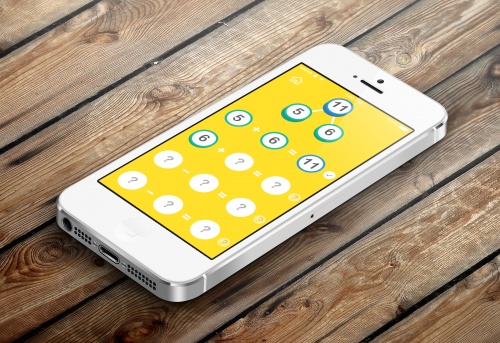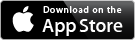Here & There · LearnTime · Maths Facts · Nuala Kawaii · Numbery · Roman Nums · Times Tables ·

# Maths FactsMaths Facts offers a series of easy to play games designed to help your child practice and learn the addition and subtraction facts, along with facts families and number bonds. Visit the app store to find out more.### Features:

• easy to play games with sliding tiles;
• colourful and animated;
• same app works on iPad and iPhone/iPod;
• different games that focus on the Number Bonds, Facts Families, as well as adding, subtracting, multiplying, and dividing;
• choose between number bonds up to 10 or up to 20.

### Games

Number Bonds – what numbers add up to 4? 0+4=4, 1+3=4, and 2+2=4.

Facts Families – what addition and subtraction facts can you make with the numbers 1, 2, and 3? The addition facts are 1+2=3 and 2+1=3. The subtraction facts are 3-1=2 and 3-2=1.

Subtraction Facts – 10 questions that test you on your subtracting.

Multiplication Facts – 10 questions that test you on your multiplying.

Division Facts – 10 questions that test you on your dividing.

Missing Addends – 10 questions that test you on finding the missing number in a sum.